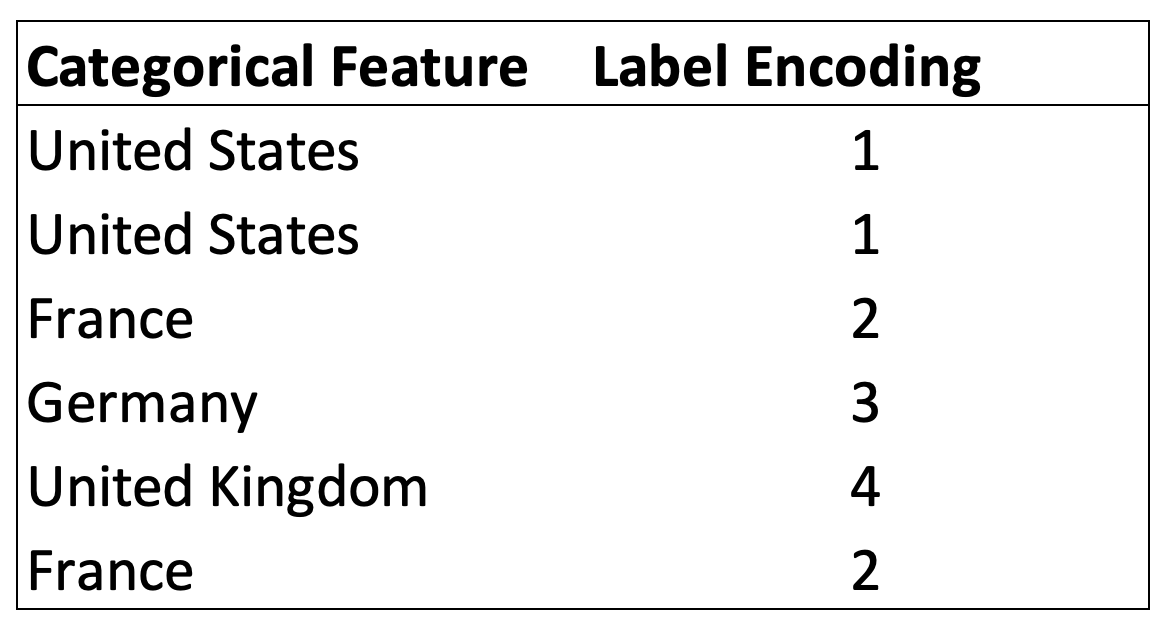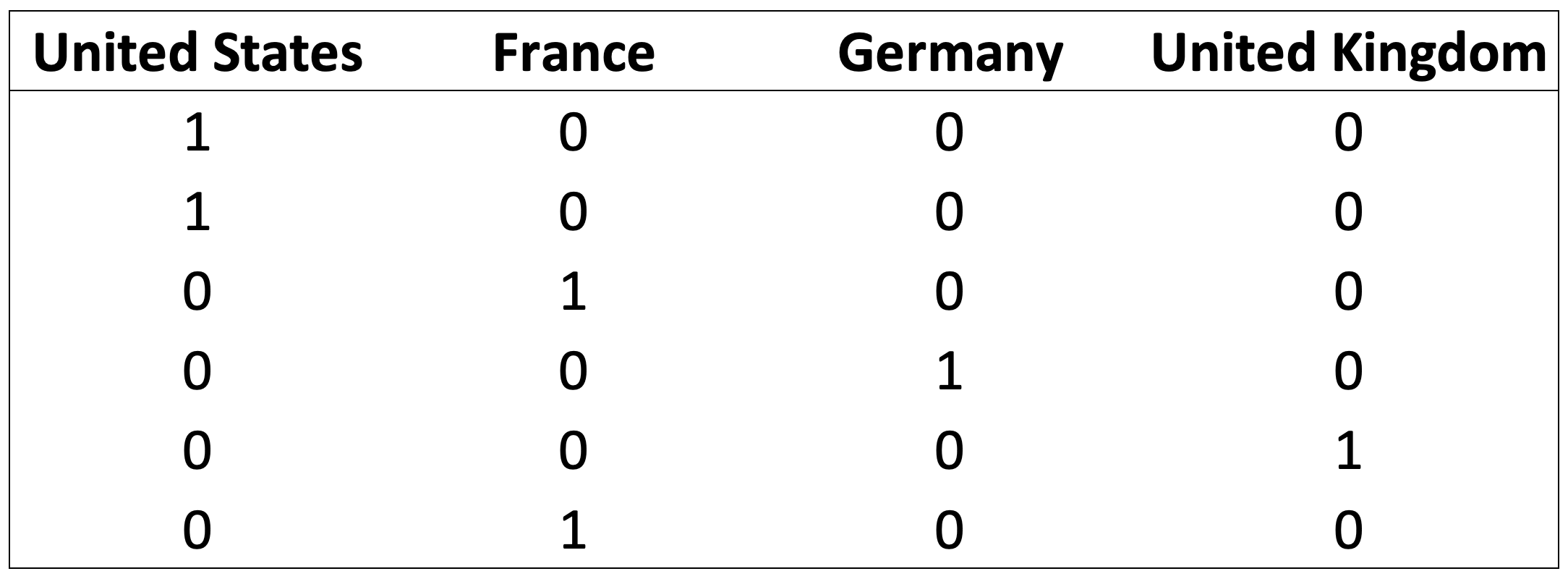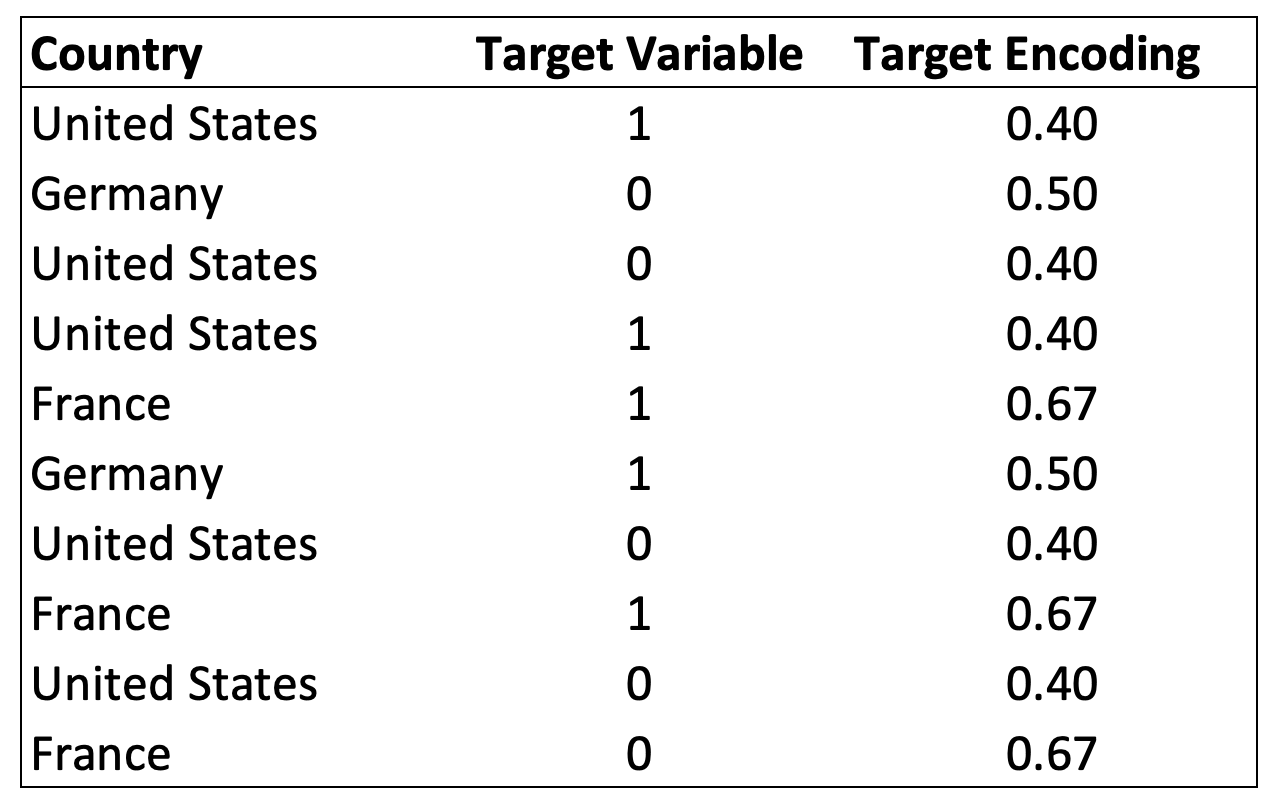# Handling Categorical Data, the right way### Handling Categorical Data, The Right Way

Categorical data is simply information aggregated into groups rather than being in numeric formats, such as GenderSex or Education Level. They are present in almost all real-life datasets, yet the current algorithms still struggle to deal with them.

Take, for instance, XGBoost or most SKlearn models. If you try and train them with categorical data, you’ll immediately get an error.

Currently, many resources advertise a wide variety of solutions that might seem to work at first, but are deeply wrong once thought through. This is especially true for non-ordinal categorical data, meaning that the classes are not ordered (As it might be for Good=0, Better=1, Best=2). A bit of clarity is needed to distinguish the approaches that Data Scientists should use from those that simply make the models run.

### What Not To Do: Label Encoding

One of the simplest and most common solutions advertised to transform categorical variables is Label Encoding. It consists of substituting each group with a corresponding number and keeping such numbering consistent throughout the feature.Example of Label Encoding

This solution makes the models run, and it is one of the most commonly used by aspiring Data Scientists. However, its simplicity comes with many issues.

### Distance and Order

Numbers hold relationships. For instance, four is twice two, and, when converting categories into numbers directly, these relationships are created despite not existing between the original categories. Looking at the example before, United Kingdom becomes twice France, and France plus United States equals Germany.

Well, that’s not exactly right…

This is especially an issue for algorithms, such as K-Means, where a distance measure is calculated when running the model.

### Solutions

#### One-Hot Encoding

One-Hot Encoding is the most common, correct way to deal with non-ordinal categorical data. It consists of creating an additional feature for each group of the categorical feature and mark each observation belonging (Value=1) or not (Value=0) to that group.Example of One-Hot Encoding

This approach is able to encode categorical features properly, despite some minor drawbacks. Specifically, the presence of a high number of binary values is not ideal for distance-based algorithms, such as Clustering models. In addition, the high number of additionally generated features introduces the curse of dimensionality. This means that due to the now high dimensionality of the dataset, the dataset becomes much more sparse. In other words, in Machine Learning problems, you’d need at least a few samples per each feature combination. Increasing the number of features means that we might encounter cases of not having enough observations for each feature combination.

#### Target Encoding

A lesser known, but very effective way of handling categorical variables, is Target Encoding. It consists of substituting each group in a categorical feature with the average response in the target variable.Example of Target Encoding

The process to obtain the Target Encoding is relatively straightforward and it can be summarised as:

1. Group the data by category
2. Calculate the average of the target variable per each group
3. Assign the average to each observation belonging to that group

This can be achieved in a few lines of code:

`encodings = data.groupby('Country')['Target Variable'].mean().reset_index()data = data.merge(encodings, how='left', on='Country')data.drop('Country', axis=1, inplace=True)`

Alternatively, we can also use the category_encoders library to use the TargetEncoder functionality.

Target Encoding is a powerful solution also because it avoids generating a high number of features, as is the case for One-Hot Encoding, keeping the dimensionality of the dataset as the original one.

### Summary

Handling categorical features is a common task for Data Scientists, but, often, people do not exactly know what are the best practices to correctly tackle them.

For non-ordinal categories, Label Encoding, which consists of substituting a category with a relatively random integer, should be avoided at all costs.

Instead, One-Hot-Encoding and Target Encoding are preferable solutions. One-Hot Encoding is probably the most common solution, performing well in real-life scenarios. Target Encoding is a lesser-known but promising technique, which also keeps the dimensionality of the dataset consistent, improving performance.